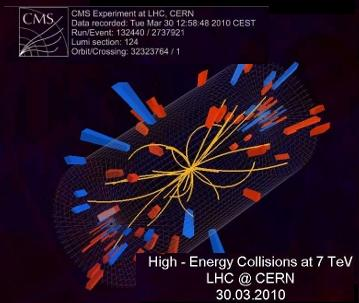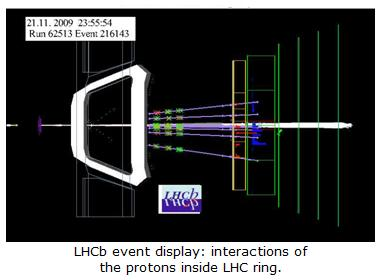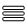Taking a closer look at LHC

The so-called “Beam Lifetime” (ζ) is the time interval after which the intensity of the beam has reached 1/e of its initial value (e is Euler’s number).

We are going to estimate this value by considering any of the more important situations wich can cause decreasing of the number of protons

First we consider the collisions among protons in the interaction points. The total proton-proton cross section at 7 TeV is approximately 110 mbarns (milibarns)

(with 1 barn = 10-24 cm2= 10-28 m2).The total collision rate at nominal luminosity is:

Nevent/s = L·σ event

1034 x [(110 x10-310-24] ~ 10collisions/second

With 2808 bunches:

109/2808    3.6·105 collisions per bunch and second.

We can consider these collisions as a proton “decay” process with a probability, λ :

Since initially the total number of protons per bunch is  Np0 ~ 1.15·1011 protons:

λ  = 3.6·05/1.15·1011     λ = 3·10-6 s-1

This value represents the probability that one proton collide with an opposing proton per second.

So, the variation of the number of protons is given by:

dNp/dt = – λ × Np

So,

Np(t) = Np0 × e-λ× t

where Np(t) is the number of protons per bunch as a function of time and Np0 the initial number of protons per bunch.

If we now solve the last equation with  Np(t) /Np0 = 1/e  and  t = ζ   we get:

1/e = e-λ× ζ

and finally,

ζ =1/λ

With  λ = 3·10-6 s-1 , we obtain:

ζ = 3·105 s (~ 80 h)

So, by considering only protons collisions in the interactions point the Beam Lifetime would be over 80 hours.

A second important process which decreases the number of protons is the the inelastic scattering produced by proton-gas collisions (see Ideal Gases Equation section). Elastic interactions are not considered because in this process the kinetic energy of the proton is conserved, and magnetic multipoles act to correct these deviations by focussing the bunch.

The main gases are H2, CH4, CO, CO2, H2O and nobles gases, but we will consider that all molecules are H2 (In fact, for calculation the rest of molecules "are converted into" H2equivalent, by introducing corrector parameters).For proton-hydrogen nucleus collision (p-H1) at 7 TeV, the inelastic scattering cross-section  is ~ 40 mb; therefore, for proton-hydrogen molecule (p-H2) this value is σ ~ 80 mb (cross-section,  σ, for proton-gas collisions represents a hypothetical area which describes the probability of a proton being scattered by a hydrogen molecule. See more ...).

σ ~ 80 mb  ⇒  σ ~ 80·10-3 x 10-28   ⇒  σ ~ 8·10-30 m2

For this calculation, we consider the 2.45-km-long LHC arcs, where the gas density (ρm) has significant importance ( see Ideal Gases Equation section).

In this case  ρm  1.4·1015 molecules/m3

The distance of the eight 2.45-km-long is  d = 8 × 2450  ⇒  d ~ 2·104 m

Then, for a single bunch (Np ~ 1011 protons) circulatingthe number (Nlap) of bunch-gas interactions per lap over a distance d  is:

Nlap ~  σ× ρ× N× d

Then,

Nlap ~ 8·10-30 × 1.4·1015  × 1.15·1011 × 2·104

Nlap ~ 26 collisions/lap  per bunch

Since bunches do 11245 laps per second (), the rate of bunch-gas interactions (Rint) is:

Rint Nlap × f

Rint ~ 26 × 11245 ~ 2.9·105  (290 kHz)

So we have around 3·105 lost protons per bunch in a second when beams begin to circulate around the LHC.

We can consider again this situation as a proton “decay” process with a probability, λ :

λ = (2.9·105)/(1.15·1011)

λ = 2.5·10-6 s-1

This value represents the probability that any proton collide against any gas molecule per second.

Using again,

ζ =1/λ

we finally obtain:

ζ = 4·105 s (~ 110 h)

So, taking only into account protons-gas collisions the Beam Lifetime would be over 110 hours.

By considering the both two process together, the Beam Life time will be:

1/ζ = 1/ζ1 + 1/ζ2

1/ζ ~ 1/80 + 1/110

ζ ~ 45 h

Other mechanisms such as limited efficiency of the correction and focalization systems,Coulomb scattering of protons travelling together (Touschek effect included), or travelling in differents bunches when crossing in interaction points, or operating errors, also contribute in decreasing the Beam Lifetime.

Due to this, finally the Beam Lifetime for the LHC is about 10 hours.

 AUTHORS Xabier Cid Vidal, PhD in experimental Particle Physics for Santiago University (USC). Research Fellow in experimental Particle Physics at CERN from January 2013 to Decembre 2015. Currently, he is in USC Particle Physics Department ("Ramon y Cajal", Spanish Postdoctoral Senior Grants). Ramon Cid Manzano, secondary school Physics Teacher at IES de SAR (Santiago - Spain), and part-time Lecturer (Profesor Asociado) in Faculty of Education at the University of Santiago (Spain). He has a Degree in Physics and in Chemistry, and is PhD for Santiago University (USC). CERN CERN WEBSITE CERN Directory CERN Experimental Program Theoretical physics (TH) CERN Physics Department CERN Scientific Committees CERN Structure CERN and the Environment LHC LHC Detector CMS Detector ATLAS Detector ALICE Detector LHCb Detector TOTEM Detector LHCf Detector MoEDAL

IMPORTANT NOTICE

For the bibliography used when writing this Section please go to the References Section

© Xabier Cid Vidal & Ramon Cid - rcid@lhc-closer.es  | SANTIAGO (SPAIN) |